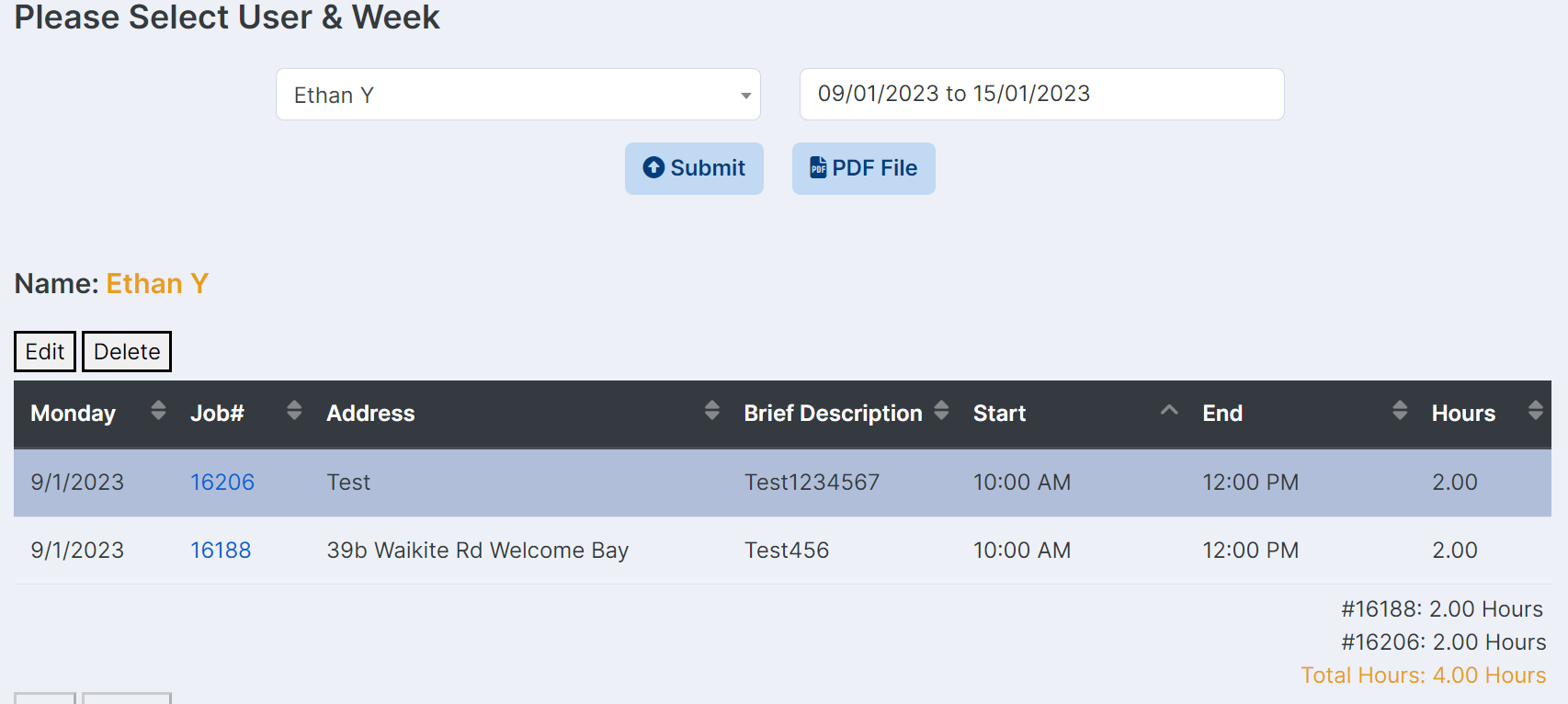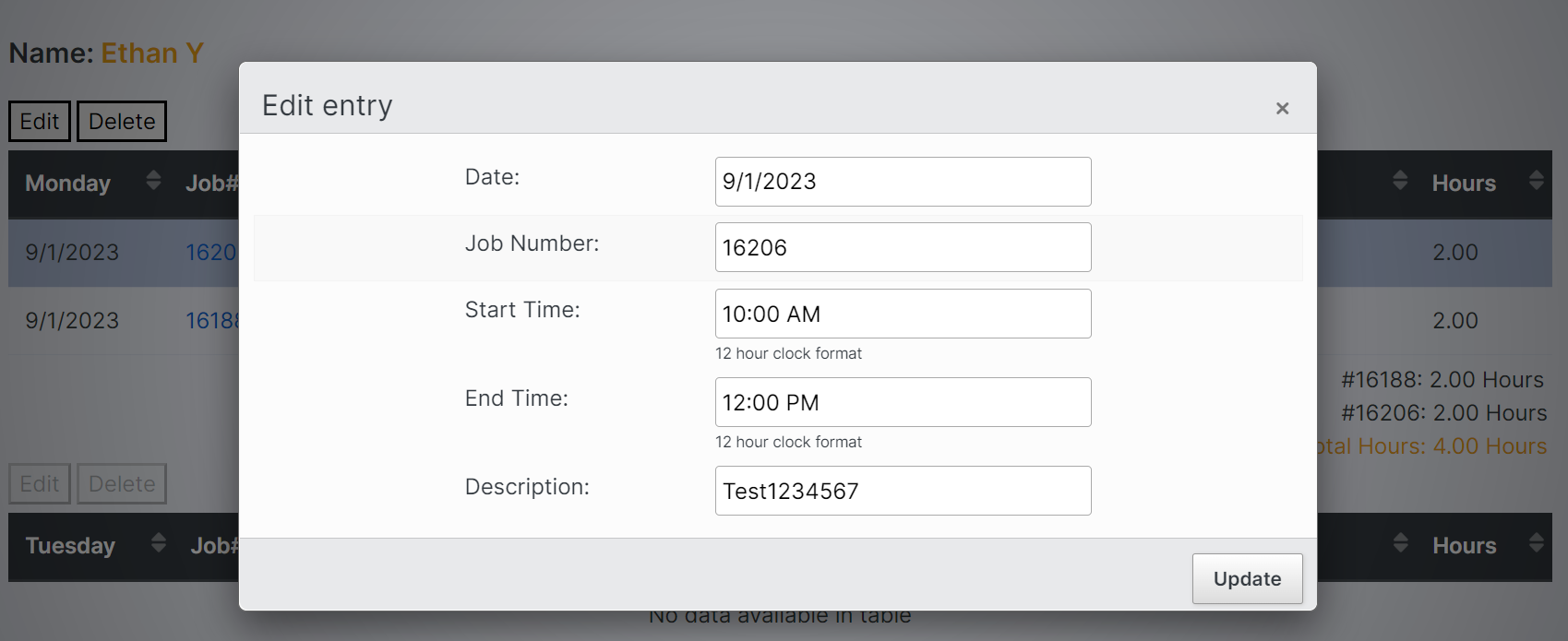# How to edit one field in Editor and another field change and match automatically?

Hi team, I have a data table and editor that can display job number and address and etc.
Job number and address are matching with each other.
When I enter the data, I used Ajax to match job number and address.
Now, I would like to edit job number and address with editor, but I cannot edit address manually because I might get a typo.
So, what I want is that only edit job number so the address can automatically change too, matching the corresponding job number. It looks like need to read database again. Could you please advise how should do it? Thank you very much!

``````Editor::inst(\$db, TBL_TIMESHEET)
->fields(
Field::inst('date'),
Field::inst('job_number'),
Field::inst('job_id'),
->setFormatter(function () {
return "Test";
}),
Field::inst('start_time')
->validator(Validate::dateFormat(
'g:i A',
ValidateOptions::inst()
->allowEmpty(false)
))
->getFormatter(Format::datetime('H:i:s', 'g:i A'))
->setFormatter(Format::datetime('g:i A', 'H:i:s')),
Field::inst('end_time')
->validator(
Validate::dateFormat(
'g:i A',
ValidateOptions::inst()
->allowEmpty(false)
),
)
->getFormatter(Format::datetime('H:i:s', 'g:i A'))
->setFormatter(Format::datetime('g:i A', 'H:i:s'))
->validator(function (\$val, \$data, \$opts) {
if (strtotime(\$val) <= strtotime(\$data['start_time'])) {
return "End time must be later than start time.";
}
return true;
}),

Field::inst('hours')
->setFormatter(function (\$val, \$data, \$opts) {

\$startTime = strtotime(\$data['start_time']);
\$endTime = strtotime(\$data['end_time']);
\$timeDif = \$endTime - \$startTime;
\$hours = \$timeDif;
\$hours = \$hours / 60 / 60;
return \$hours;
}),
Field::inst('description'),
)
->where('user_full_name', \$selectedUser, '=')
->where('date', \$weekStartDay)
->debug(true)
->process(\$_POST)
->json();
``````It sounds to me like this would be an ideal situation for a left join. Assuming you have a "jobs" table that contains the job id and other information such as an address (which I guess you must do since you mention doing a lookup to get the new address from the new job number).

That way, you would left join to `jobs` based on the job id, and get the address, thus it would be updated automatically when the form is submitted. There is an example of a left join here.

Is that an option for you?

If not, and you need to duplicate the address in the timesheet table, then you would need to query the database to get the new address. That would be done in a server-side event (`preCreate` and `preEdit`).

Allan

• edited January 15

Hi Allan, thank you for your help.
Yes I have two tables: jobs and timesheet.
But unfortunately I need to duplicate the address in the timesheet table~
Because it is a old project, the data base was not designed very well.
Other parts of the app need to read address in Timesheet Table~~~
I have seen the server-side event you gave, but could not figure out how to query database. I have not been very familiar with the APIs of Editor yet.
This is what I have done so far, but looks like it obviously wrong~
(I also added Select2 to job_number this time)
I think I don't need to creat connection myself~ But have not found a way how to do it.
And also, need to get the new address with the new job_number in Jobs Table and replace the old address with the new address in Timesheet Table.
So confused

``````    Editor::inst(\$db, TBL_TIMESHEET)
->fields(
Field::inst('date'),
Field::inst('job_number'),
Field::inst('job_id'),
->setFormatter(Format::ifEmpty(null))
->options(
Options::inst()
->table(TBL_TIMESHEET)
->value('job_number')
->label('job_number')
->render(function (\$row) {
return html_entity_decode(\$row['job_number']);
})
)
->validator(Validate::dbValues()),
// ->setFormatter(function () {
//     return "Test";
// }),
Field::inst('start_time')
->validator(Validate::dateFormat(
'g:i A',
ValidateOptions::inst()
->allowEmpty(false)
))
->getFormatter(Format::datetime('H:i:s', 'g:i A'))
->setFormatter(Format::datetime('g:i A', 'H:i:s')),
Field::inst('end_time')
->validator(
Validate::dateFormat(
'g:i A',
ValidateOptions::inst()
->allowEmpty(false)
),
)
->getFormatter(Format::datetime('H:i:s', 'g:i A'))
->setFormatter(Format::datetime('g:i A', 'H:i:s'))
->validator(function (\$val, \$data, \$opts) {
if (strtotime(\$val) <= strtotime(\$data['start_time'])) {
return "End time must be later than start time.";
}
return true;
}),

Field::inst('hours')
->setFormatter(function (\$val, \$data, \$opts) {

\$startTime = strtotime(\$data['start_time']);
\$endTime = strtotime(\$data['end_time']);
\$timeDif = \$endTime - \$startTime;
\$hours = \$timeDif;
\$hours = \$hours / 60 / 60;
return \$hours;
}),
Field::inst('description'),
)
->where('user_full_name', \$selectedUser, '=')
->where('date', \$weekStartDay)
->on('preEdit', function (\$editor, \$id, \$values) {
\$editor
->setValue(
function () {
\$connection = new PDO('mysql:host=' . DB_SERVER . ';dbname=' . DB_NAME, DB_USER, DB_PASS);
\$connection->setAttribute(PDO::ATTR_ERRMODE, PDO::ERRMODE_EXCEPTION);

\$query = "SELECT a.address FROM " . TBL_JOBS . " a LEFT JOIN " . TBL_TIMESHEET . " b ON a.job_number = b.job_number";
\$stmt = \$connection->prepare(\$query);
\$stmt->execute();
\$result = \$stmt->fetch(PDO::FETCH_ASSOC);

}
);
})
->debug(true)
->process(\$_POST)
->json();
``````
• I made it！I read the old code in the project I am maintaining.

``````    ->on('preEdit', function (\$editor, \$id, \$values) {
\$getAddress = \$editor->db()->sql('SELECT address FROM ' . TBL_JOBS . ' WHERE job_number = ' . \$values['job_number'])->fetch();
})
``````

But could not found these method, db(), setValue(), select(), in the Editor's manual.
Are these PHP method or Editor's ?

Thank you team!

• They are part of the Editor PHP libraries. Overview documentation for them are here, while the proper details are here in the PHP API reference (along with the rest of the PHP API for Editor).

Great to hear you got it done!

Allan